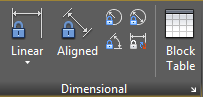# BCPARAMETER (Command)

Applies constraint parameters to selected objects, or converts dimensional constraints to parameter constraints.The BCPARAMETER command is used in the Block Editor. This command applies a constraint parameter to an object or between constraint points on object(s).

The following prompts are displayed.

### Linear

Find

Creates a horizontal or vertical constraint parameter based on the locations of the extension line origins and the location of the dimension line similar to the DIMLINEAR command.

Object

Selects an object instead of a constraint point. Press Enter or click the drop-down list to select the object.

### Horizontal

Find

Constrains the X distance of a line or between two points on different objects. Valid objects include lines and polyline segments.

Object

Selects an object instead of a constraint point. Press Enter or click the drop-down list to select the object.

### Vertical

Find

Constrains the Y distance of a line or between two points on different objects. Valid objects include lines and polyline segments.

Object

Selects an object instead of a constraint point. Press Enter or click the drop-down list to select the object.

### Aligned

Find

Constrains the length of a line or the distance between two lines, a point on an object and a line, or two points on different objects.

Object
Object
Point & Line

Selects a point and a line object. The aligned constraint controls the distance between a point and the closest point on a line.

2Lines

Selects two line objects. The lines are made parallel and the aligned constraint controls the distance between the two lines.

### Angular

Find

Constrains the angle between two lines or polyline segments. It is similar to an angular dimension.

3Point

Selects three valid constraint points on the object.

 Valid Objects or Points Characteristics Pair of lines Pair of polyline subobjects Three constraint points Arc When you select two lines, the angle between the lines is constrained. The initial value always defaults to a value less than 180 degrees. When you specify three constraint points, the following applies: First point — angle vertex Second and third points — endpoints of the angle When you select an arc, a three-point angular constraint is created. The angle vertex is at the center of the arc and the angle endpoints of the arc are at the endpoints of the arc.

When you enter or edit an angle value that is either negative or is greater than 360 degrees, the number entered is stored for the expression (for example, 390), but the value displayed is based on the formatting of the units (for example, 30 if decimal degrees).

When an expression with variables evaluates to greater than 360 or less than -360, the constraint value is displayed in the Parameters Manager palette based on the units of the drawing.

Find

Creates a radial constraint parameter for a circle, arc, or polyline arc segment.

Valid Object or Points Characteristics
Circle Constrains the radius of the circle or arc.
Arc

### Diameter

Find

Creates a diameter constraint parameter for a circle, arc, or polyline arc segment.

Valid Object or Points Characteristics
Circle Constrains the diameter of the circle or arc.
Arc

### Convert

Find

Converts the dimensional constraints to constraint parameters.

Objects other than dimensional constraints are ignored and filtered from the selection set.